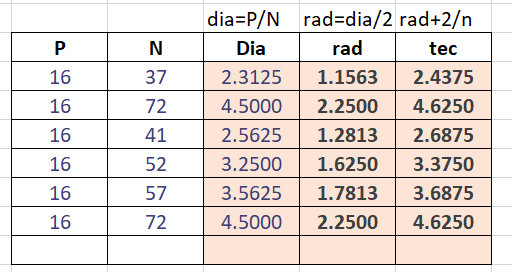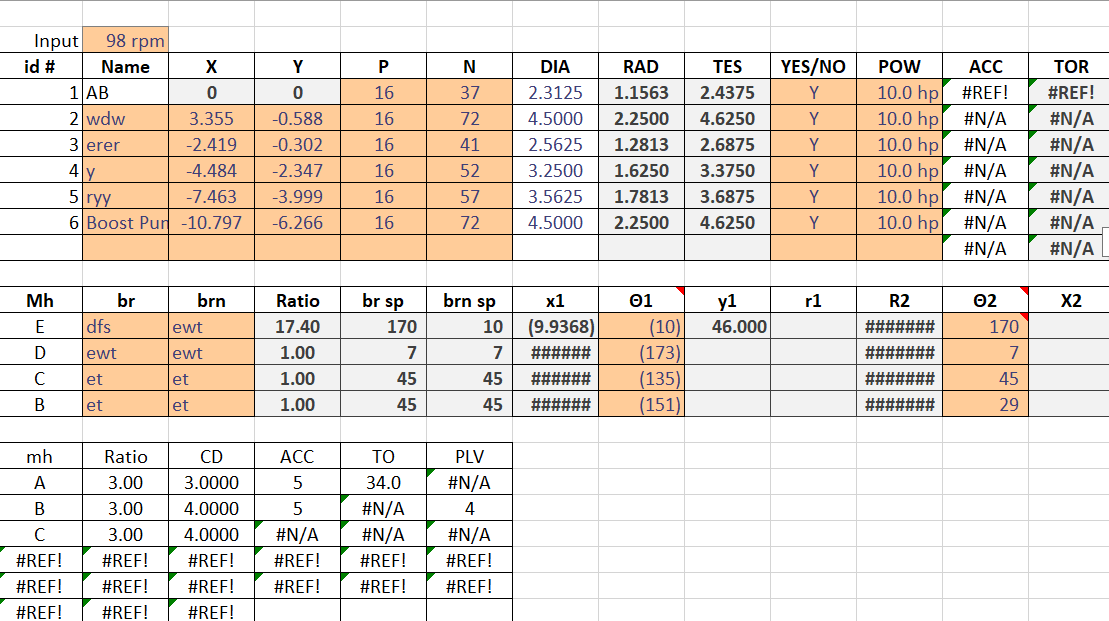# Qtableview calculation

• Anyone can share the qtableview code or any example
where any calculation is implemented on columns.
like if user enter in column 1 and 2 so in column 3 result will display. and like that

• Override data() in your model and return the sum of col1 and col2 for col3 for Qt::DisplayRole

• @Christian-Ehrlicher actually this i am asking for example purpose i have to implement different formula in columns not only one

• @n-2204 said in Qtableview calculation:

this i am asking for example purpose

I don't know what example you want here. When you've your own model you also have to implement your own data() function where you can return whatever you want for every cell.

• As i am new i dont understand how to implement in my model.
so to implement i need to understand how to do/ create functions.

i need to implement some formula not just add in columns so automatic calculation will happen when user enter data in columns

• When you don't have an own model yet you should start with https://doc.qt.io/qt-5/model-view-programming.html and it's examples.

• @Christian-Ehrlicher i have models
model = new QStandardItemModel(7, 13, this);//row*col
model2 = new QStandardItemModel(7, 20, this);
model3 = new QStandardItemModel(7, 10, this);
but the calculaion part i dont understand how to implement

• @n-2204 said in Qtableview calculation:

but the calculaion part i dont understand how to implement

• @Christian-Ehrlicher can i get any related examples from any site ?
one approach is data() change using QAbstractTableModel()
and i have 3 model so how to do in that case?

QVariant calculationm::data( const QModelIndex &index, int role ) const
{
switch( role )
{
case Qt::DisplayRole:
}

• @n-2204 said in Qtableview calculation:

can i get any related examples from any site ?

There are enough on this side... to lazy to search?
No more comments from me here.

if i able to found then no need to ask here.
and i have written the approach also how to do

• You can use `KExtraColumnsProxyModel`: https://api.kde.org/frameworks/kitemmodels/html/classKExtraColumnsProxyModel.html

``````#include <QApplication>
#include <QStandardItemModel>
#include <QTableView>
#include <KExtraColumnsProxyModel>

class ExampleCalcProxy : public KExtraColumnsProxyModel{
Q_DISABLE_COPY(ExampleCalcProxy)
public:
explicit ExampleCalcProxy(QObject* parent = nullptr)
: KExtraColumnsProxyModel(parent)
{
// the first extra column will sum the column 0 and 1
appendColumn(tr("Sum"));
// the second extra column will multiply the column 0 and 1
appendColumn(tr("Product"));
}
QVariant extraColumnData ( const QModelIndex &  parent, int  row, int  extraColumn, int  role = Qt::DisplayRole) const override{
if (role != Qt::DisplayRole && role != Qt::EditRole)
return QVariant();
const double column0data = index(row,0,parent).data(role).toDouble();
const double column1data = index(row,1,parent).data(role).toDouble();
switch (extraColumn) {
case 0:
return column0data+column1data;
case 1:
return column0data*column1data;
default:
return QVariant();
}
}
};

int main(int argc, char *argv[])
{
QApplication a(argc, argv);
// create a model with 2 columns
//and 5 rows and fill it with random numbers
QStandardItemModel model;
model.insertColumns(0,2);
model.insertRows(0,5);
for(int i=0;i<5;++i){
for(int j=0;j<2;++j){
model.setData(model.index(i,j),rand()%100);
}
}
// instantiate the proxy and set the source model
ExampleCalcProxy proxy;
proxy.setSourceModel(&model);
// usa a table view to display the result
QTableView view;
view.setModel(&proxy);
view.show();
return a.exec();
}
``````

•i need to implement the table formula for column i added at column
i have created tableview using model
there are 3 qtableview
model = new QStandardItemModel(7, 13, this);//row*col
model2 = new QStandardItemModel(7, 20, this);
model3 = new QStandardItemModel(7, 10, this);
now how can i implement calculation in a separate cpp file
how can i do this ? i am new to Qt and c++ please suggest

• @n-2204 said in Qtableview calculation:

there are 3 qtableview

No, there is one `QTableView` shown. There seem to be 3 models. Why do you have 3 separate models? What you seem to have is one model, with 2 columns of input data (`P` & `N`) and 3 columns of calculated data. Unless you have some requirement I don't understand, that is what you want. That is also the principle employed in @VRonin's example.

•There seem to be 3 models.
Why do you have 3 separate models? What you seem to have is one model, with 2 columns of input data (`P` & `N`) and 3 columns of calculated data

colored column will be user input and other column have some formula when use enter data so other column should update accordingly
if for one table i implement then i understand how to do so i just shared one table picture
so i dont understand the implementation of formula
. Unless you have some requirement I don't understand, that is what you want.